# Methods of valuation of goodwill | Accounts Class 12

Methods of Valuation of Goodwill

Methods of Valuation of Goodwill, which  are most commonly used are as under : –

(i) Average profit method of valuation of goodwill

(ii) Super profit method of valuation of goodwill

(iii) Capitalisation method of valuation of goodwill
(a)Capitalisation of Average Profit
(b)Capitalisation of Super Profit

In order to understand the methods of Valuation of Goodwill, it is important to understand the meaning of Number of Year’s Purchase. Generally, the goodwill represents the ability of a company to earn certain excess profits over the normal profits earned by competitors for certain number of years. Number of Year’s Purchase, implies that the firm is expected to earn the same amount of profits , as they are earning, after the admission (or retirement/death) of a new partner for the given number of years .

### Methods of valuation of goodwill –  1  – Average Profit Method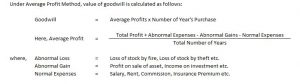Goodwill – Average Profit Method – Question 1 : –

Aaroh and Avroh were partners in a firm. They admit Anant for 3/4 the share in partnership, on 1st April 2016. Calculate the value of Goodwill on the basis of 4 year’s purchases of normal average profits of the last 5 years. The Profit and Loss Account showed the profit as follows: ”

2012-60,000 , 2013-75,000 , 2014-90,000 , 2015-75,000 , 2016-1,05,000

Explanation : –

Goodwill = Average profit X Numbers of year’s purchase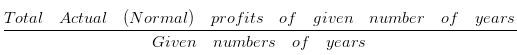Total number of years = 5
Number of year purchase = 4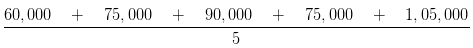= 405000/5

= 81,000

Goodwill = Average profit X Numbers of year’s purchase
= 81,000 X 4
= 3,24,000 Rs.

Goodwill – Average Profit Method –  (Abnormal Gain & Abnormal Losses)

Question : –

A and B were partners in a firm .They admit C for 1/3rd share in profit on 1st January 2015 .Value of the Goodwill is to be calculated based on the basis of 2 year’s purchases of normal average profits of last 5 years. The Profit and Loss Account showed the profit as follows:
2010-Rs.5,000 Including an abnormal gain of Rs 1,000
2011-Rs.10,000 Excluding Rs 4,000 as insurance premium
2012-Rs.4,000 After charging an abnormal loss of Rs 3,000
2013-Rs.8,000
2014-Rs.2,000 Loss
The value of goodwill will be:

Explanation : –

Goodwill = Average profit X Numbers of year’s purchaseTotal number of years = 5
Number of year purchase = 2
Average Maintainable profits
Years
2010 = 5,000 – 1,000 = 4,000
2011 = 10,000 – 4,000 = 6,000
2012 = 4,000 + 3,000 = 7,000
2013 = 8,000
2014 = -2,000
Rs. 23,000

Average profit = 23000/5

= 4,600
Goodwill = Average profit X Numbers of year’s purchase
= 4,600 X 2
= 9,200 Rs.

##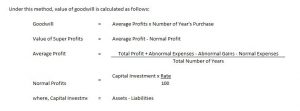Goodwill – Super  Profit Method – Question 1 : –

A and B who were partners in a firm admitted C for 1/4 share of profits on 1st April 2015. The capital investment of the firm was Rs. 1,00,000. A fair return on the capital, having regard to the risk involved is 10 %. If the profits for the last 2 years were 2013 = 40,000 Rs. , 2014 = 50,000 Rs. Find the value of goodwill on the basis of 2 year purchase of super profit?

Explanation : –

Goodwill = Super profit X Numbers of year’s purchase
Super Profit = Average Profit – Normal profit

Average profit =Given numbers of years
Total number of years = 2
Number of year purchase = 2

Average profit = (40000+50000+0)/2

= 90000/2

= 45,000
Normal profit = Capital investment X Normal rate of return

= 1,00,000 X 10/100

= 10,000
Super Profit = 45,000 – 10,000
= 35,000
Goodwill = 35,000 x 2
= Rs. 70,000

### 3. Methods of valuation of goodwill –  3 – Capitalisation Method :-

Under this method, valuation of goodwill can be calculated by capitalisation of average profit or capitalisation of super profit.

#### Methods of valuation of goodwill –  3a  – Capitalisation of Average Profit Method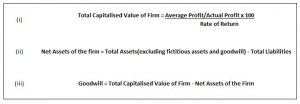Goodwill – Capitalization of Average Profit Method –

Question 1 : –

A firm earns Rs. 1,00,000 as its annual profit, the normal rate of profit being 5 %.Assets of the firm are Rs. 15,00,000 excluding goodwill and liabilities are Rs. 5,00,000 .The value of goodwill by capitalisation method will be:

Explanation : –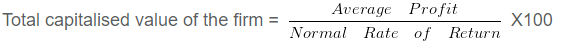= 100000/5 X100

= 20,00,000
Net asset of the firm = Total Assets – Liabilities
= 15,00,000 – 5,00,000
= 10,00,000
Goodwill = Total capitalised value of business – Net assets
= 20,00,000 – 10,00,000
= Rs. 10,00,000

#### Methods of valuation of goodwill –  3b – Capitalisation of Super Profit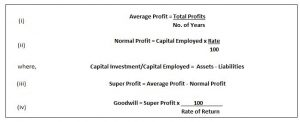Goodwill – Capitalization of Average Super Method –

Question : –

A firm having assets of Rs. 1,00,000 and liabilities of Rs. 1,50,000 earns the annual profits of Rs. 45,000 .The rate of normal profit being 10 %.The amount of goodwill by capitalisation of super profits will be:

Explanation : –

Average profits = 45,000
Capital employed (Net assets) = 1,00,000 – 1,50,000
= -50,000= -50,000 X 10/100

= -5,000

Super profits = Average profits – Normal profits

= 45,000 – (-5,000)
= 50,000= 50000/10 X 100

= Rs. 5,00,000

Weighted Average Profit Method is the method of computing goodwill, where value of Goodwill is equal to the (Weighted Average Profit X Number of year’s purchase). Weighted Average Profit are calculated as under : –

• Assign  given weights to the profits of the respective years. Generally, higher weights are given to recent year profits.
• Multiply profits of respective year with the weights;
• Add all the Products so obtained
• Divide the sum of Products by number of years to get the Weighted Average Profit

Goodwill = Weighted Average Profit X Number of year’s purchase

Some examples of methods of valuation of goodwill are as given below:

Goodwill – Average profit method-Insurance Premium

Question : –

A and B were partners in a firm .They admit C for 1/2 share in profit on 1st January 2016 Compute Average maintainable profit, for the Year 2017, if there was an insurance premium of Rs. 6000 excluded from profit of Rs. 17,000 . The value of goodwill will be:

Explanation : –

Average Maintainable profits = Profit for the Year – Insurance premium
= 17,000 – 6,000
= 11,000

Goodwill – Average Profit Method(Type 4)

Question : –

A and B were partners in a firm .They admit C for 2/3 share in profit on 1st January 2016 .Compute Average maintainable profit, for the Year 2017, if there was an abnormal loss of Rs. 3,000 included in the total profit of Rs. 10,000 . The value of goodwill will be:

Explanation : –

Average Maintainable profits = Profit for the Year + Abnormal Loss
= 10,000 + 3,000
= 13,000 Rs.

Goodwill – Capitalisation of Super Profit(Type 2)

Question : –

Average profit of the firm are Rs. 2,00,000 .Total tangible assets in the firm are Rs . 25,00,000 and Outside Liabilities are Rs. 10,00,000 .In the same type of business , the normal rate of return is 10 % of the capital employed.The value of goodwill by capitalisation of super profit method will be:

Explanation : –

Capital Employed = Total Tangible Assets – Outside Liabilities
= 25,00,000 – 10,00,000
= 15,00,000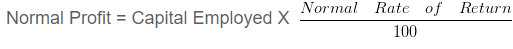= 15,00,000 X 10/100

= 1,50,000

Super Profit = Average Profit – Normal Profit

= 2,00,000 – 1,50,000
= 50,000

###= 50000/10 X 100

= Rs. 5,00,000

Goodwill – Normal Average Profit Method

Question : –

B , C and D are partners sharing profits and losses in the ratio of 2 : 1 : 3 . They admit E for 1/3 share. For the purpose of admission of E ,the goodwill of the firm is to be valued on the basis of 1 years purchase of last 3 or 4 years profits,whichever is higher. The profits were: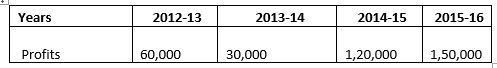The value of goodwill will be:

Explanation : –

Calculation of Average profits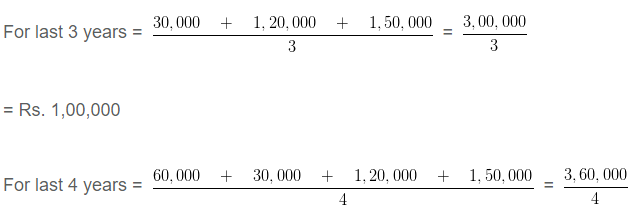=Rs.  90,000
Since the profit of Year 3 are higher, goodwill shall be calcualted based on the profit of 3 th Year
Goodwill = Average proft of 3 years X Number of year purchase
= 1,00,000 X 1
= Rs. 1,00,000

Goodwill – Super profit Method(Type 3)

Question : –

Average net profit of A Ltd expected in the future is Rs . 100000 per year.The average capital employed in the business is Rs 500000 .Normal profit expected from capital invested in this class of business is 10 %.The Remuneration of the partners is estimated to be Rs 20000 per annum.The value of goodwill on the basis of 3 year’s purchase of super profit will be :

Explanation : –

Average Annual Profit = 1,00,000 Rs.
Less : Remuneration = -20,000 Rs.
Actual Profit = 80,000 Rs.

Normal profit on capital employed = 5,00,000 X 10/100 = 50,000

Annual super profit = 80,000 – 50,000
= 30,000
Goodwill = 30,000 X 3
= Rs. 90,000

1.